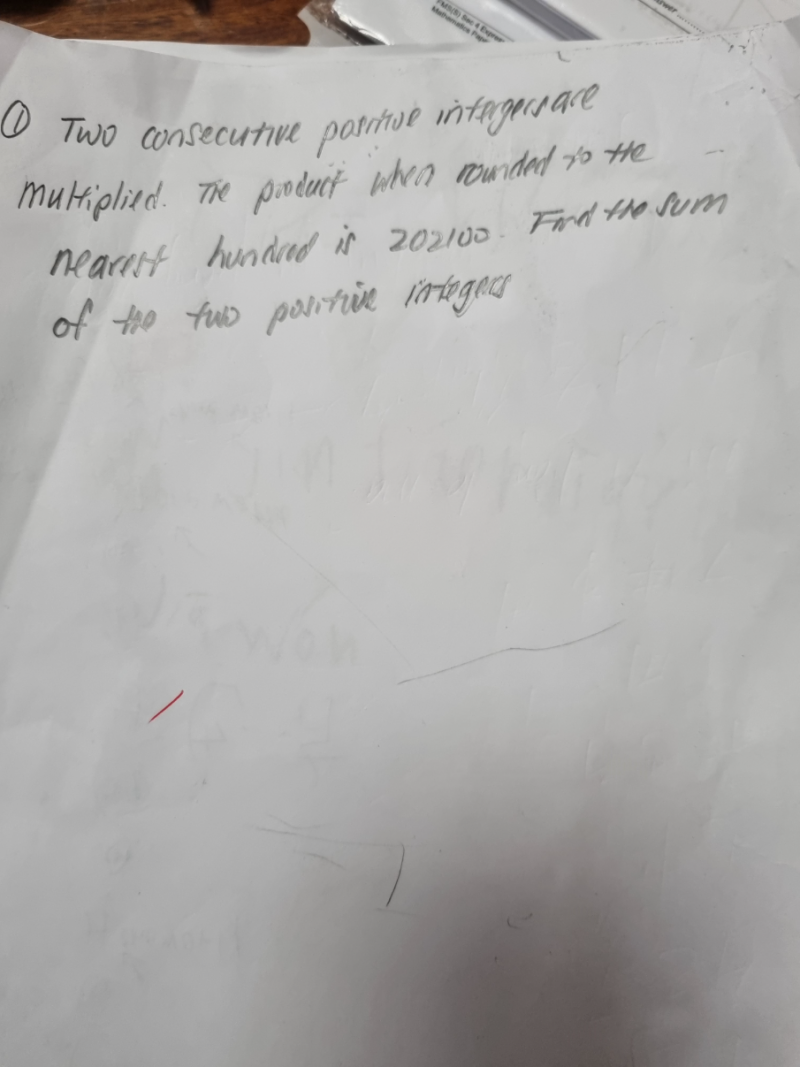# QuestionPls help thank you.

I used estimation reasoning for this question and guess and check.

Since 500 x 500 = 250 000   (this is important to know where to start the guess and check)

it means the two positive numbers is  less than 500 but likely greater than 450.

So I did guess and check with 450 x 450 = 202 500 and the range has been established.

So the numbers should be between 448-451

448 x 449 = 201 152  (this is rounded  as 201 200 to the nearest 100)

449 x 450 = 202 050 (this is rounded as 202 100 to the nearest 100)

Hence sum of the 2 positive integers = 449 + 450 = 899

0 Replies 2 Likes ✔Accepted Answer